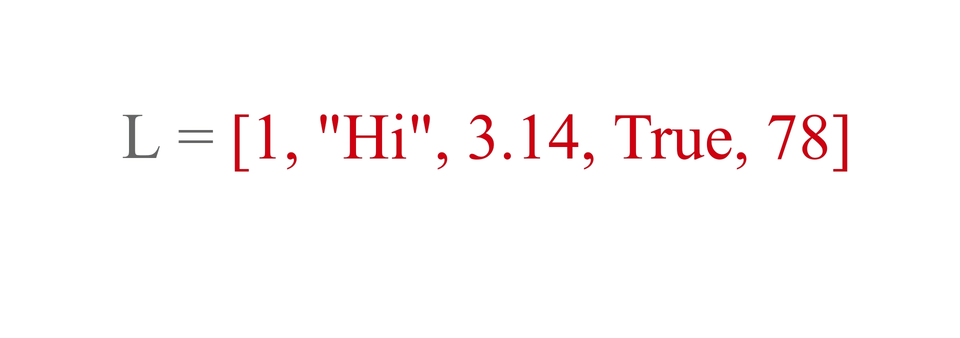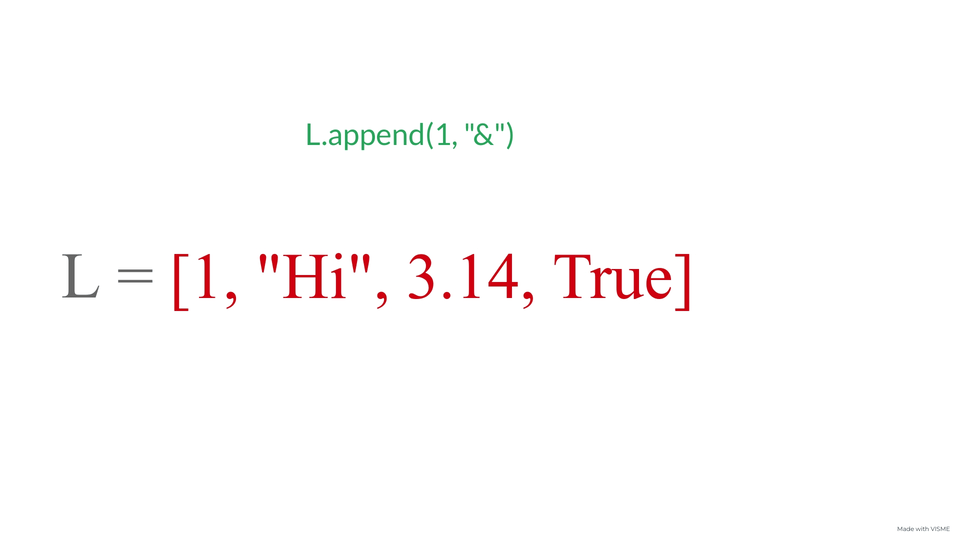# Lists in PythonCreated February 26, 2022

List is a data structure used in Python. It is similar to the concept of arrays but unlike arrays they're more flexible about the type of data that is being stored in them. It is very easy to work with lists as their implementation and operations are very easy to learn and remember.

The first question that comes to our mind while learning a new concept in a language is how we can implement it. Being a coder, it is very necessary for us to be able to check how a certain piece of code might work and experiment with it. So whenever you come across a new concept, just try to play with it for a while and use it in different ways to know better about it.## Representation

It is very easy to represent a list in Python. It is basically represented by square brackets '[ ]'. All the elements inside a list are comma(,) separated. Note that elements of a list can be of any data type.

`List_name = [element 1, element 2,...element n];`

Following is an example for the same -

``````l = [1,"hi", 'a', 6.3]
``````

...and that's it!## Index

Each element in a list is assigned a number called "Index" which is used to identify its position in the list. In programming languages, the index values start from 0 and goes on up to n-1(n being the number of elements in the list).

``````l = [1,"hi", 'a', 6.3]
#    0   1    2    3   : index values
``````

So if for instance you want to access the element that's present at a certain index poisition, you can simply do so in the following way -

`list_name[index position]`

Eg-

``````l = [1,"hi", 'a', 6.3]
#    0   1    2    3   : index values
a = l
``````

Here variable "a" stores the value present at index 1 in list "l". If you want to print it directly from the list, you can simply just do this -

``````l = [1,"hi", 'a', 6.3]
#    0   1    2    3   : index values
print(l)
``````Easy...isn't it?

## Insertion

### Insert at a specific index position

If you have a certain value that has to inserted in a list at a certain position, you can simply use the "insert" function. It is an in-built function and very easy to use. Following is the format for using insert operation on a list. `List_name.insert(index_position, element value)`

A code snippet for the same -

``````l = [1,"hi", 'a', 6.3]
#    0   1    2    3   : index values
l.insert(1, 80)
``````

Now, if you try to access the element at index=1, then it'll return 80 and similarly if you try to access the element present at index=2, it'll return "hi". Why?

``````l = [1,"hi", 'a', 6.3]
#    0   1    2    3   : index values
l.insert(1, 80)
print("Element at index=1: "+str(l)+" element at index=2: "+l)
``````

That happened because, the list elements on and after 1st element got shifted backward by 1 position to that the new element can be added to the index position 1.### Insert at the end of the list

The word Append is basically used to act of adding something at the end. In Python we use "append" function to append or insert an element at the end of the list. So, if you want to add something at the end of a list you can do that like this -

``````l = [1,"hi", 'a', 6.3]
#    0   1    2    3   : index values
l.append("last")
print(l)
``````

## Modifying list elements

Ok, so till now we learned about how we can represent a list and how to insert elements into it. But what if you want to modify the value present at a certain index position? You can do that by reassigning value stored at that index, like this -

``````l = [1,"hi", 'a', 6.3]
#    0   1    2    3   : index values
l = 6
``````

Adding one list with another list is very much possible in python, you simply have to use "+" operation between the two lists to add them. eg:

``````l1 = ["a", 1, True]
l2 = ["b", 2, True]
l3 = l1+l2
print(l3)
``````

So as you can see, it literally adds the list l1 first and then list l2 into list l3.

You can also multiply lists with integer values. Try for yourself and find out what happens!

## Conclusion

It very simple to work with lists in Python. The main advantage of lists in python over arrrays in any other language is that you can have any data type inside a list whereas you can have only single data type elements in an array. It makes our work all the more easier!

I hope you liked reading this article and got a bit of idea about how you can use lists in python. For more articles in Data Structures and Algorithm you can visit this link.